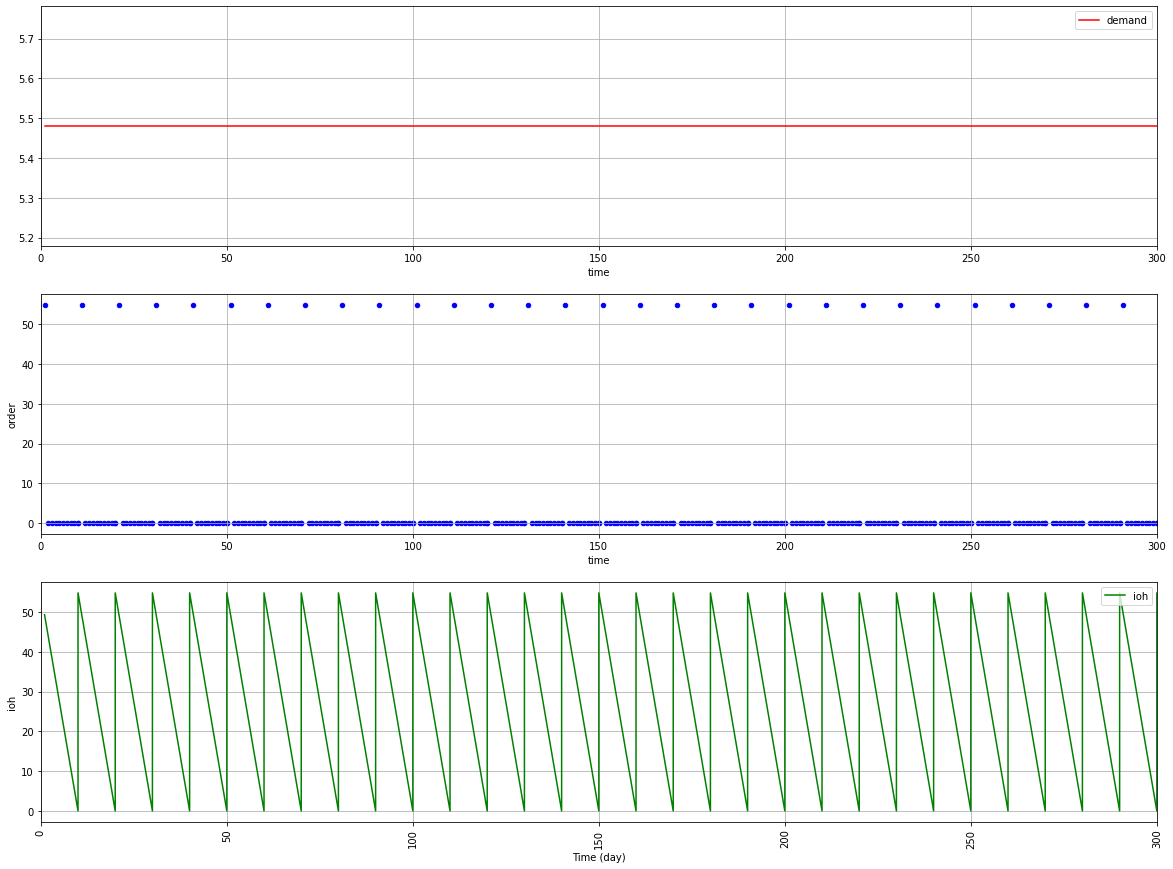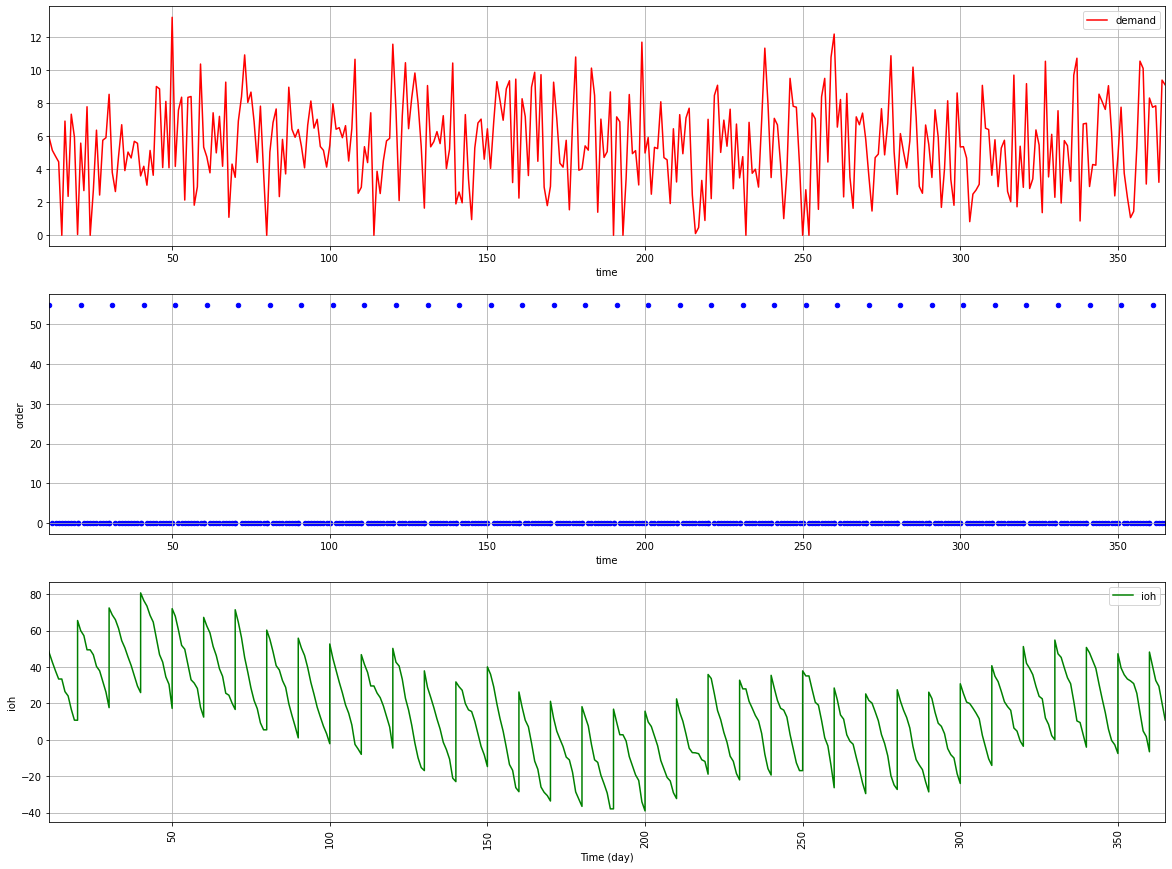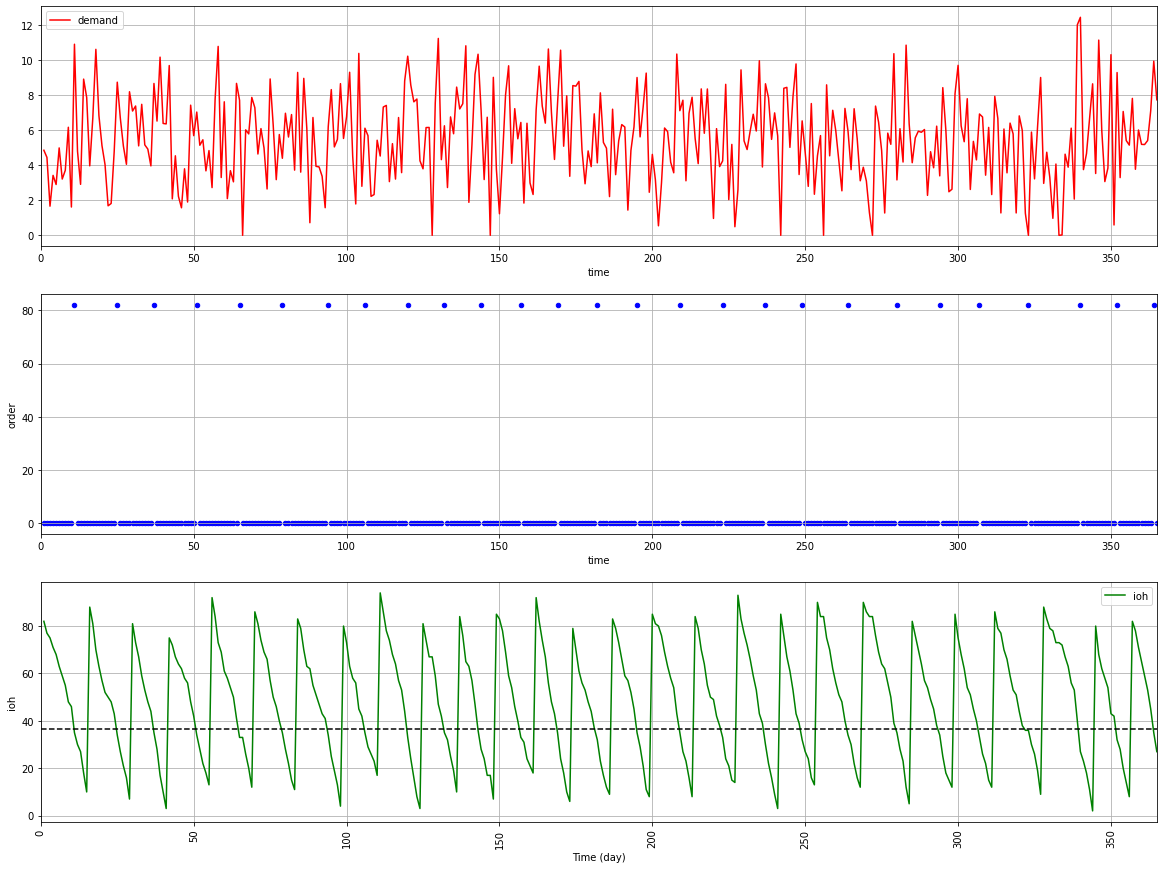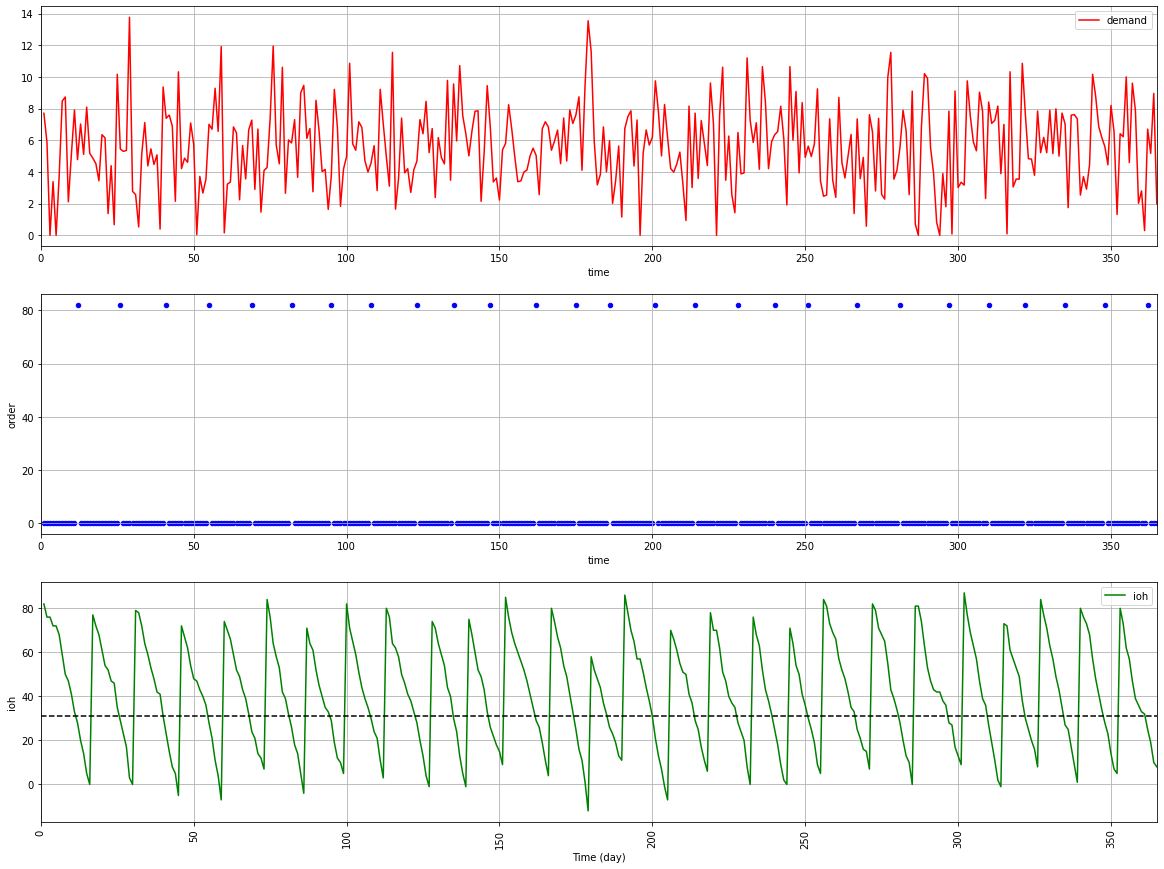# Inventory Management for Retail — Stochastic Demand

Simulate the impact of safety stock level on inventory management performance metrics assuming a normal distribution of your demand

Simulate the impact of safety stock level on inventory management performance metrics assuming a normal distribution of your demand

Article originally published on Medium.

For most retailers, inventory management systems take a fixed, rule-based approach to forecast and replenishment orders management.

Considering the distribution of the demand, the objective is to build a replenishment policy that will minimize your ordering, holding and shortage costs.

In a previous article, we have built a simulation model assuming a deterministic constant demand (Units/Day).

In this article, we will improve this model and introduce a simple methodology using a discrete simulation model built with Python to test several inventory management rules assuming a normal distribution of the customer demand.

💡
SUMMARY
I. Scenario
1. Problem Statement
As an Inventory Manager of a mid-size retail chain, you are in charge of setting the replenishment quantity in the ERP.
2. Limits of the deterministic model
What could be the results with a normally distributed demand?
II. Continuous Review Policy: Order Point, Order Quantity (s, Q)
1. Introduction of the Inventory Policy
2. Definition of the Safety Stock
3. How do you define k?
III. Example of replenishment policies
1. Target of CSL = 95%
2. Target of IFR = 99%
III. Conclusion & Next Steps

## I. Scenario

### 1. Problem Statement

As an Inventory Manager of a mid-size retail chain, you are in charge of setting the replenishment quantity in the ERP.

Based on the feedback of the store manager, you start to doubt that the replenishment rules of the ERP are the most optimal especially for the fast runners because your stores are facing lost sales due to stock-outs.

For each SKU, you would like to build a simple simulation model to test several inventory rules and estimate the impact on:

Performance Metrics

• Cycle Service Level (CSL): probability to have a stock-out for each cycle (%)
• Item Fill Rate (IFR): percentage of customer demand met without stock-out (%)

💡
# Total Demand (units/year)
D = 2000
# Number of days of sales per year (days)
T_total = 365
# Customer demand per day (unit/day)
D_day = D/T_total
# Purchase cost of the product (Euros/unit)
c = 50
# Cost of placing an order (/order)
c_t = 500
# Holding Cost (% unit cost per year)
h = .25
c_e = h * c
# Selling Price (Euros/unit)
p = 75
# Lead Time between ordering and receiving
LD
# Cost of shortage (Euros/unit)
c_s = 12
# Order Quantity
Q = 82 (units/order)

To simplify the comprehension, let’s introduce some notations

### 2. Limits of the deterministic model

In the previous article, we assumed a constant deterministic demand; we’ll now introduce randomness to get closer to real demand.Initial model with constant demand D = 5.4 units/day — (Image by Author)
What could be the results with a normally distributed demand?

µ_D = 2000 (items/year)
σ_D = 50(items/year)Initial model with Stochastic Yearly Demand Distribution N(2000, 50) — (Image by Author)
You need to improve your replenishment policy to compensate for the volatility of your demand.
🔗
You can find the full code in my Github repository: Link

## II. Continuous Review Policy: Order Point, Order Quantity (s, Q)

### 1. Introduction of the Inventory Policy

To solve this issue of demand volatility, we’ll introduce a continuous review policy (s, Q)

• Continuous Review = your inventory level will be checked every day
• (s, Q) = if your inventory level ≤ s your ERP will order Q

To simplify the comprehension, let’s introduce some notations:

### 2. Definition of the Safety Stock

The reorder point can be defined as the minimum inventory level you need to meet your customers’ demand during the lead time between your ordering and receiving.

The safety stock is a buffer to compensate for the volatility of the demand.

### 3. How do you define k?

Your performance metrics will be directly impacted by the safety stock level; the highest k is the best your performance will be:

1. You fix your target for any of the two metrics (e.g: I want my CSL to be 95%)
2. You calculate k to reach this target
3. You fix your reorder point

## III. Example of replenishment policies

### 1. Target of CSL = 95%

Based on the definition of the CSL, we have:

k = 1.64
Reoder point with CSL: 36 units(s, Q) model with s = 32 units— (Image by Author)

In this example, we can see that we do not face any stock and the minimum stock level is very close to zero.

Code

### 2. Target of IFR = 99%

In this previous example, our target was to have 95% of the replenishment cycles without stock-out.

In this example, we’ll focus more on our capacity to deliver products in full with a target of IFR.

# G(k) = Q/sigma_ld * (1 - IFR)
IFR = 0.99
G_k = (Q/sigma_ld) * (1 - IFR) = 0.14# Final value of k
k = 0.71
Reoder point with CSL: 31 units(s, Q) model with s = 31 units — (Image by Author)

To reach 99% of demand units fulfilled without stock-out you need a lower safety stock. (31 units vs. 32 units)

Code

## IV. Conclusion and next steps

### Conclusion

This improved model is bringing better results as it considers the variability of the demand in the safety stock sizing.

The process is simple, you start by fixing your performance metrics targets (IRF, CSL) and then you calculate your safety stock level using the k value.

### Next Steps

The main issue with the continuous review policy is the high number of replenishment if you have many SKUs in your portfolio.

As a store manager (or Warehouse Manager), you would prefer to fix the replenishment time (e.g: 2 times per week). Therefore, we will introduce the periodic review policy in the next article.